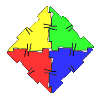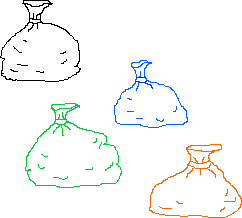#### You may also like### Geoboards

This practical challenge invites you to investigate the different squares you can make on a square geoboard or pegboard.### Polydron

This activity investigates how you might make squares and pentominoes from Polydron.If you had 36 cubes, what different cuboids could you make?

# Money Bags

## Money Bags

Ram divided $15$ pennies among four small bags.He labelled each bag with the number of pennies inside it.

He could then pay any sum of money from $1$p to $15$p without opening any bag.

How many pennies did Ram put in each bag?

This problem is based on Money Bags from 'Mathematical Challenges for Able Pupils Key Stages 1 and 2', published by DfES. You can find out more about this book, including how to order it, on the Standards website here .

### Why do this problem?

This problem is a good example of a challenge which does not require high-level mathematics, but does need a systematic approach.  It also lends itself to a focus on different ways of recording.

### Possible approach

In order that children understand the requirements of this problem, it would be a good idea to begin by reversing the situation. Draw two bags on the board and label them $1$p and $3$p. Invite children to suggest the amounts you could pay using these bags if you are not allowed to open either of them [$1$p, $3$p and $4$p]. Introduce another bag to go with the first two which contains $5$p. This time, ask the children which amounts of money they wouldn't be able to make using these bags [$2$p, $7$p, anything of $10$p and above]. You could give them time in pairs to work on this, perhaps using a mini-whiteboard to make jottings.

Invite pairs to share their solutions and highlight those that have used a systematic approach, for example by starting with the smallest amount of money and working up in steps of a penny at a time. You can then introduce the problem as it stands. Give pairs chance to work together before once again sharing their ideas. Before they give the answer, ask children to explain how they went about finding the solution.

The whole class could then check the solution offered by trying to make all the different amounts from $1$p to $15$p.  You may also wish to share different ways of recording used by various pupils.  You could discuss the advantages of each method and it would be worth asking whether children would change their chosen method if, for example, they were told someone else was going to read it.  This gives you an opportunity to highlight the fact that we might record differently, depending on what the recording is for and who is going to see it.

### Key questions

How will you make $1$p?
How will you make $2$p? ...
How will you make sure you can pay for all the amounts from $1$p to $15$p?

### Possible extension

You could challenge children to extend this problem by asking what amount they would need in a fifth bag to be able to make as many amounts as possible over $15$p.

### Possible support

Having equipment available might help some children grasp this problem. Try to let them choose what they would like to use, but having some small bags handy might be a good idea.  Encouraging them to try out ideas is vital.